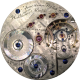Home / Database / What is the PWDB Relative Rarity Index?

# What is the PWDB Relative Rarity Index?

/
5883 ViewsExample of the PWDB Relative Rarity Index for the Elgin Grade 91Now displayed on most serial number lookups on the Pocket Watch Database

## Overview

The PWDB Relative Rarity Index is a statistical metric representing the rarity of a particular grade within the context of the total estimated serialized production from a single company.

This index value aims to quantify relative rarity within a watch company’s production, independent of the absolute rarity that modern collectors often discuss.

All rarity index values are calculated within the context of grade, size, model, and configuration classification and then normalized using standard deviation. The variation with the lowest production (the “rarest” grade/size/model/configuration variation produced by the company) will receive a PWDB Relative Rairty Index value of 0. Variations representing the company’s average production total will receive a PWDB Relative Rairty Index value of 100. Thus, values 0-100 will be assigned to variations with estimated total production units fewer than the average for the company, representing movements that would be considered “rarer than average” relative to the company’s total production.

Variations produced in quantities exceeding the average for the factory will be assigned PWDB Relative Rairty Index values greater than 100. Due to the statistical calculation method, this value has no upper limit. The higher the metric, the more common the variation production.

## Examples:

In most cases, Elgin grade classifications encompass the size, model, and configuration – making these an ideal example study. With an estimated total production of only 50, the Elgin Grade 38 is considered the rarest standard grade produced at the Elgin factory. As a result, this grade will assume a rarity index value of zero.

#### Grade 38 PWDB Relative Rarity Index: 0.00

(Estimated Total Production: 50)

Many collectors are familiar with the 21-Jewel Interchangeable grades that are highly collectible due to the low production and prestigious finishing. The Grade 91 and 72 would be assigned the following rarity index values:

#### Grade 91 PWDB Relative Rarity Index: 1.11

(Estimated Total Production: 350)

#### Grade 72 PWDB Relative Rarity Index: 2.27

(Estimated Total Production: 450)

As shown, these index values are still very close to 0. Grades that have low index values and premium features will typically be more collectible.

When accounting for all Elgin serialized production, the Grade 335 represents the “average” total production for a targeted variation. As a result, this grade would be assigned an index value of 100.00. All index values below 100 imply an estimated output of fewer than 86,000 units (“rarer than average”). Conversely, all values above 100.00 reflect an estimated total production greater than 86,000 units (“more common than average”).

#### Grade 335 PWDB Relative Rarity Index: 100.00

(Estimated Total Production: 86,000)

Elgin’s Grade 303 was the most popular serialized grade produced at the Elgin factory. With a total estimated production of over 2 million units, this grade would dictate a massive index value relative to average production.

### Grade 303 PWDB Relative Rarity Index: 2577.02

(Estimated Total Production: 2,215,000)

This index value represents a total production quantity over 10 standard deviations from the average. The large index value indicates a huge disparity with what would be considered “normal” production output. A high PWDB Relative Rarity Index value suggests the grade is very common and, as a result, likely less collectible than watches with lower estimated values (though, exceptions exist).

The PWDB Relative Rarity Index is a network of interdependent statistical metrics. As a result, these values will automatically adapt to other information modified in the database. For instance, if a run previously assumed to be entirely fulfilled is discovered to be only partially fulfilled before termination, the change applied to the run will decrease the run quantity. As a result, the total production for the grade classification will also decrease, causing a correlated increase in rarity. Since the statistical metrics are interdependent, all other rarity index values will adapt according to this change.

For this reason, the PWDB Relative Rarity Index values are expected to change over time as new information becomes available. The values will also change if different segmentation scopes are applied to company production (i.e., scoping for setting or jeweling variants in addition to the master grade/size/model/configuration classification).

## Objective

The PWDB Relative Rarity Index aims to provide a quantified metric to convey how rare a particular movement is within the context of total serialized production from the factory. This index can quickly identify which movements represent common or uncommon production using real-time statistical methods to analyze relative rarity.

## Calculation Method

The PWDB Relative Rarity Index is calculated for each grade/size/model/configuration variation relative to other similarily-grouped serialized variations produced at the same factory.

The estimated total production for each variation is calculated based on the quantities represented by each serialized run, forming the core data for statistical analysis. These values are then used to calculate the mean production and standard deviation before being normalized to yield an index value.

1.Bradford Hammer says:

My Hamilton 1939 950 has a rarity index of 9.24.
Serial2631272. Is this a valuable watch?

bhammer60@gmail.com

•Nathan Moore says:

Yes. The Hamilton 950 is quite collectible.

2.R.L. Sherwood says:

My Waltham Watch company, P.S. Bartlett Grade, Model 1877 key wound pocket watch, Serial Number 1656473, produced between November – December 1881, Run Quantity: 1,000, has a PWDB Relative Rarity Index of 251.29 and a PWDB Absolute Rarity Index of 251.29.

Is this collectible? What would the average value be?

•Nathan Moore says:

A rarity index value of 100 is considered average.

3.Robert Kiefer says:

I have a Waltham pocket watch serial number 7749464. Rarity number 6.38. Is this a valuable watch?

4.Alan Holland says:

My Elgin 9kt gold pocket watch 5278699. Is it rare or worth anything.

This div height required for enabling the sticky sidebar